# Lévy-Prokhorov metric

(diff) ← Older revision | Latest revision (diff) | Newer revision → (diff)
Jump to: navigation, search

A metric in the space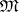of finite Borel measures (cf. Borel measure) on a metric space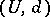, defined bywhereis the-algebra of Borel sets ofand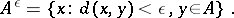The Lévy–Prokhorov metric was introduced by Yu.V. Prokhorov  as a generalization of the Lévy metric. The quantitychanges if in its definition one omits one of the two inequalities and replaces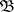by the system of all open or closed sets of(see ).

## Most important properties of the Lévy–Prokhorov metric.

1) The metric space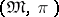is separable if and only ifis separable (cf. Separable space).

2) The spaceis complete if the spaceis complete (cf. Complete space). The converse is true if the measures ofhave separable supports.

3) In the spaceof probability measures the Lévy–Prokhorov metric has properties analogous to those of the Lévy metric. Namely, the regularity property 3) (cf. Lévy metric) and its corollaries, properties 4) and 5), property 6) (in the case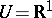), property 7) in part (namely,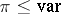), and also an analogue of property 8) ifis a linear normed space: If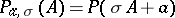, where,, then for any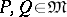,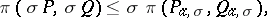4) In the case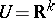the Lévy–Prokhorov metric incan be estimated by means of the characteristic functionsandcorresponding to the measuresand(see , ).

5) The Lévy–Prokhorov metric is a minimal metric with respect to the probability distancethat is, for any random variables,with fixed marginal distributions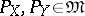one hasover all joint distributions.